Anúncio

# Continuosly compound interest and a comparison of exponential growth phenomena

16 de Aug de 2014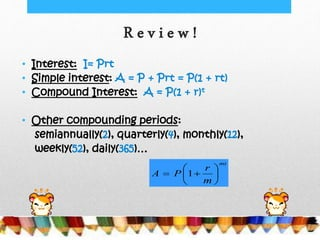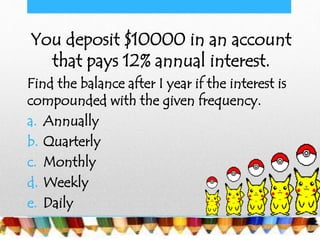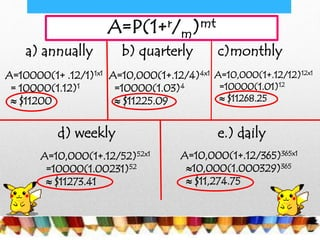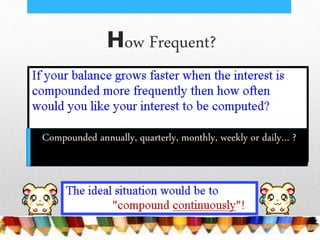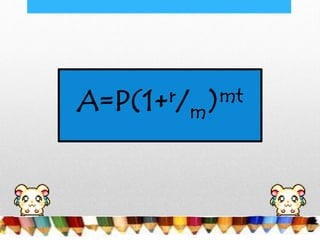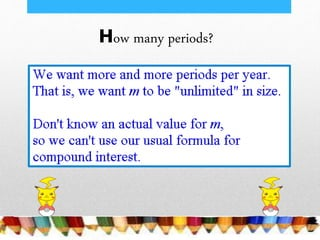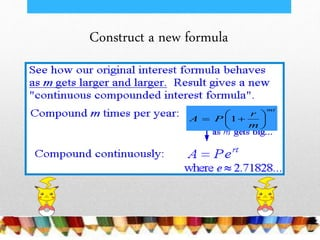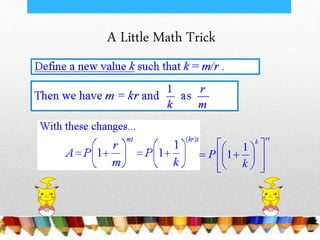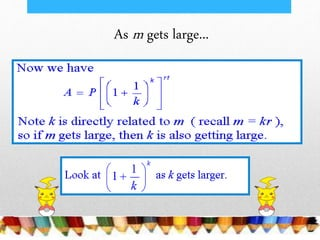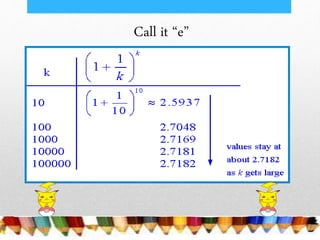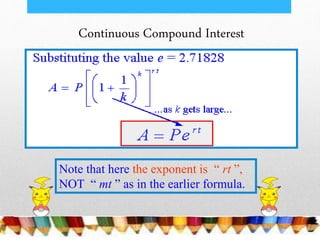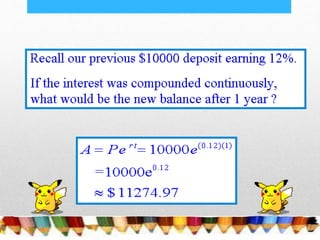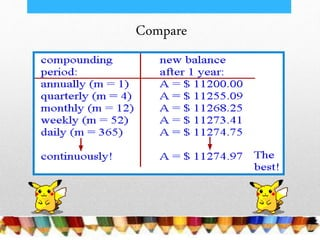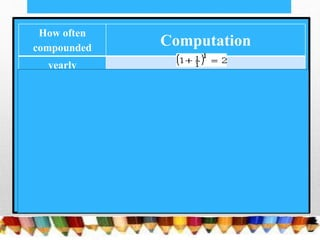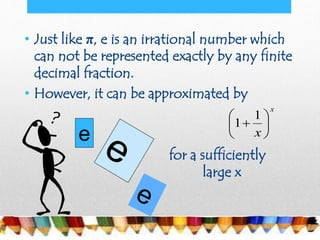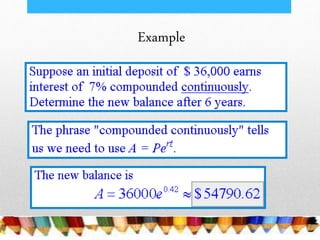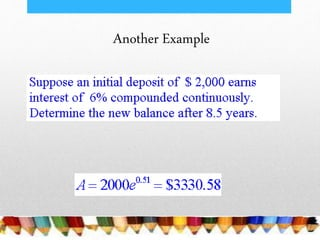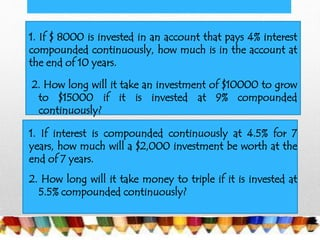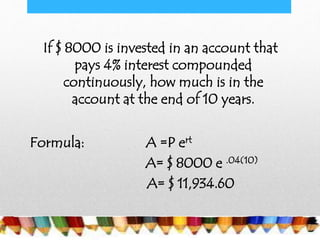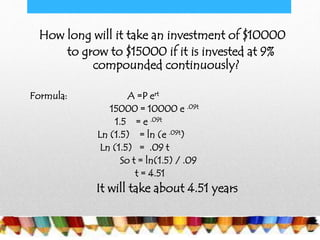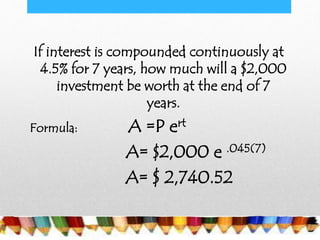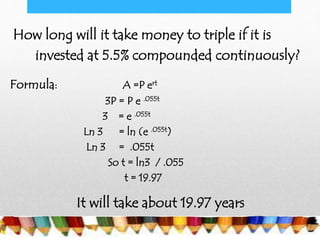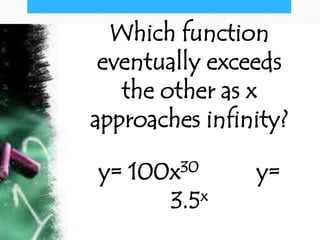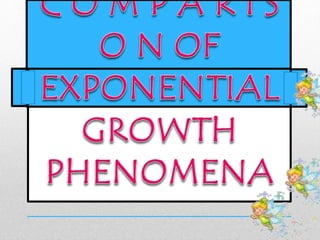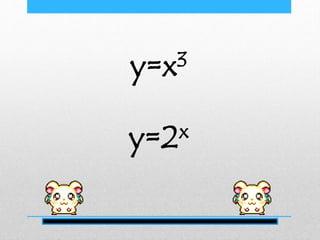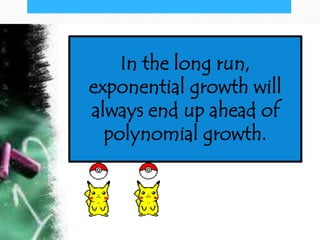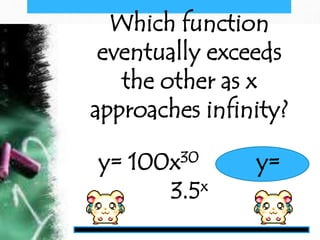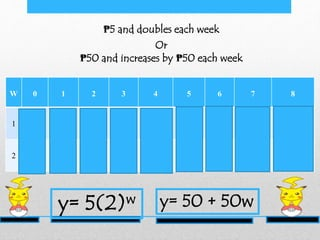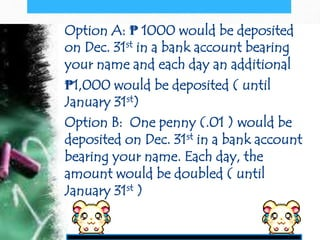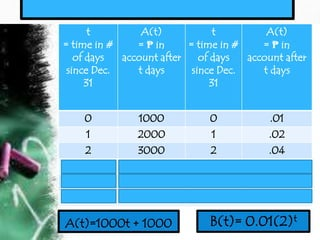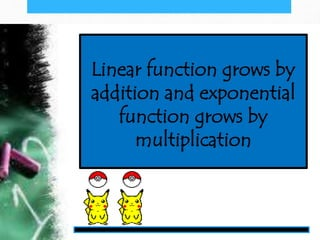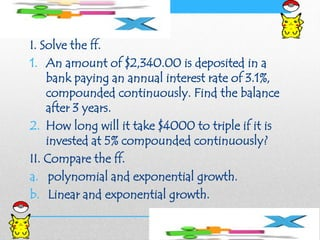1 de 39
Anúncio

### Continuosly compound interest and a comparison of exponential growth phenomena

1. R e v i e w ! • Interest: I= Prt • Simple interest: A = P + Prt = P(1 + rt) • Compound Interest: A = P(1 + r)t • Other compounding periods: semiannually(2), quarterly(4), monthly(12), weekly(52), daily(365)… mt m r PA        1
2. You deposit \$10000 in an account that pays 12% annual interest. Find the balance after I year if the interest is compounded with the given frequency. a. Annually b. Quarterly c. Monthly d. Weekly e. Daily
3. a) annually b) quarterly c)monthly d) weekly e.) daily A=10000(1+ .12/1)1x1 = 10000(1.12)1 ≈ \$11200 A=10,000(1+.12/4)4x1 =10000(1.03)4 ≈ \$11225.09 A=10,000(1+.12/365)365x1 ≈10,000(1.000329)365 ≈ \$11,274.75 A=10,000(1+.12/12)12x1 =10000(1.01)12 ≈ \$11268.25 A=10,000(1+.12/52)52x1 =10000(1.00231)52 ≈ \$11273.41 A=P(1+r/m)mt
4. How Frequent? Compounded annually, quarterly, monthly, weekly or daily… ?
5. A=P(1+r/m)mt
6. How many periods?
7. Construct a new formula mt m r PA        1
8. A Little Math Trick 1 1 rtk P k           
9. As m gets large...
10. Call it “e”
11. Continuous Compound Interest Note that here the exponent is “ rt ”, NOT “ mt ” as in the earlier formula.
12. Compare
13. How often compounded Computation yearly semi-annually quarterly monthly weekly daily hourly every minute every second
14. • Just like π, e is an irrational number which can not be represented exactly by any finite decimal fraction. • However, it can be approximated by for a sufficiently large x e x x        1 1
15. A = P e rt
16. Example
17. Another Example
18. 1. If \$ 8000 is invested in an account that pays 4% interest compounded continuously, how much is in the account at the end of 10 years. 2. How long will it take an investment of \$10000 to grow to \$15000 if it is invested at 9% compounded continuously? 1. If interest is compounded continuously at 4.5% for 7 years, how much will a \$2,000 investment be worth at the end of 7 years. 2. How long will it take money to triple if it is invested at 5.5% compounded continuously?
19. If \$ 8000 is invested in an account that pays 4% interest compounded continuously, how much is in the account at the end of 10 years. Formula: A =P ert A= \$ 8000 e .04(10) A= \$ 11,934.60
20. How long will it take an investment of \$10000 to grow to \$15000 if it is invested at 9% compounded continuously? Formula: A =P ert 15000 = 10000 e .09t 1.5 = e .09t Ln (1.5) = ln (e .09t) Ln (1.5) = .09 t So t = ln(1.5) / .09 t = 4.51 It will take about 4.51 years
21. If interest is compounded continuously at 4.5% for 7 years, how much will a \$2,000 investment be worth at the end of 7 years. Formula: A =P ert A= \$2,000 e .045(7) A= \$ 2,740.52
22. How long will it take money to triple if it is invested at 5.5% compounded continuously? Formula: A =P ert 3P = P e .055t 3 = e .055t Ln 3 = ln (e .055t) Ln 3 = .055t So t = ln3 / .055 t = 19.97 It will take about 19.97 years
23. Which function eventually exceeds the other as x approaches infinity? y= 100x30 y= 3.5x
24. y=x3 y=2x
25. X x3 2x 1 1 2 2 8 4 3 27 8 4 64 16 5 125 32 6 216 64 7 343 128 8 512 256 9 729 512 10 1000 1024
26. In the long run, exponential growth will always end up ahead of polynomial growth.
27. Which function eventually exceeds the other as x approaches infinity? y= 100x30 y= 3.5x
28. ₱50 and increases by ₱50 each week ₱5 and doubles each week Or
29. W 0 1 2 3 4 5 6 7 8 1 5 10 20 40 80 160 340 680 1,360 2 50 100 150 200 250 300 350 400 450 ₱5 and doubles each week Or ₱50 and increases by ₱50 each week y= 5(2)w y= 50 + 50w
30. Option A: ₱ 1000 would be deposited on Dec. 31st in a bank account bearing your name and each day an additional ₱1,000 would be deposited ( until January 31st) Option B: One penny (.01 ) would be deposited on Dec. 31st in a bank account bearing your name. Each day, the amount would be doubled ( until January 31st )
31. B(t)= 0.01(2)t t = time in # of days since Dec. 31 A(t) = ₱ in account after t days t = time in # of days since Dec. 31 A(t) = ₱ in account after t days 0 1000 0 .01 1 2000 1 .02 2 3000 2 .04 10 11000 10 10.24 21 22000 21 20,971.52 31 32000 31 21,474,836.48 A(t)=1000t + 1000
32. Linear function grows by addition and exponential function grows by multiplication
33. I. Solve the ff. 1. An amount of \$2,340.00 is deposited in a bank paying an annual interest rate of 3.1%, compounded continuously. Find the balance after 3 years. 2. How long will it take \$4000 to triple if it is invested at 5% compounded continuously? II. Compare the ff. a. polynomial and exponential growth. b. Linear and exponential growth.
34. Ma’am DianN:)
Anúncio